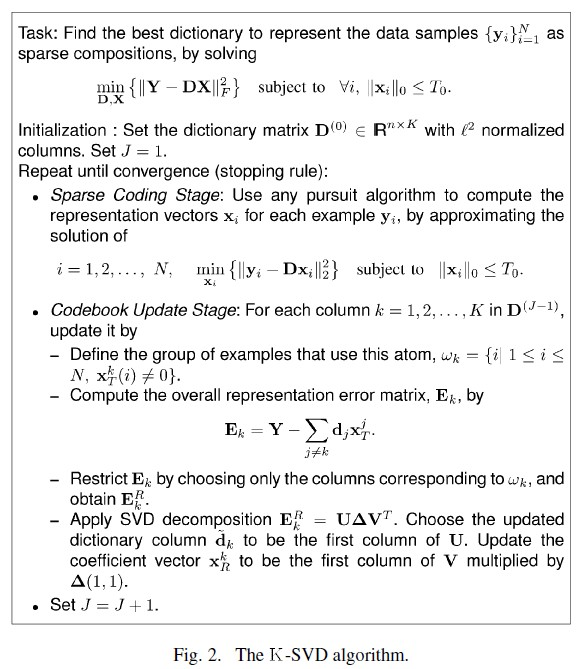# K-SVD

K-SVD 是一种关于稀疏表示的字典学习算法。之所以称之为K-SVD ，是因为该算法K次迭代使用SVD（singular value decomposition）。K-SVD是 k-means的一种推广，该算法采用迭代交替学习方式，通过迭代优化输入数据在当前字典的表示和更新字典中的单词（atom）以更好地拟合数据1] 。 K-SVD在图像处理、语音处理、生物和文件分析等众多领域被广泛应用。

给定一个过完备的字典$D \in \mathbb{R}^{n \times K}$，该字典包含$K$个单词，在此每一列都被视作一个单词。一个信号$y \in \mathbb{R}^{n}$ 能够被表示为这些单词的线性组合。为表示$y$，稀疏表示$x$ 应当满足精确条件$y = Dx$, 或者满足近似条件$y \approx Dx$, 或者满足条件$\|y - Dx\|_p \le \epsilon$. 向量$x \in \mathbb{R}^{K}$ 由表示$y$的系数组成. 通常来说, 范数$p$ 可以选为L1L2, 或者 L .$(P_0) \quad \min \limits _x \|x\|_0 \qquad \text{subject to } y = Dx$$(P_{0, \epsilon}) \quad \min \limits _x \|x\|_0 \qquad \text{subject to } \|y - Dx\|_2 \le \epsilon$

## K-SVD 算法

K-SVD 是 K-means的一种泛化。稀疏表示的目的是用少数的几个单词来表示一个样本，K-means只用一个单词表示一个样本（K-means的中心为单词。因此.  k-means能够被视作 sparse representation的一种方法. 这就是, 通过最近邻（nearest neighbor）寻找最好的可能码本表示数据样本$\{y_i\}^M_{i=1}$ 。 通过解决问题$\quad \min \limits _{D, X} \{ \|Y - DX\|^2_F\} \qquad \text{subject to } \forall i, x_i = e_k \text{ for some } k.$$\quad \min \limits _{D, X} \{ \|Y - DX\|^2_F\} \qquad \text{subject to }\quad \forall i , \|x_i\|_0 = 1.$

K-SVD 算法遵循K-means的类似条件。然而, 和 K-means不同的是， 为表示为字典D的线性组合，稀疏项的条件放宽到每列$x_i$的非零元素数目可以大于1,但是少于$T_0$。因此，目标函数变为$\quad \min \limits _{D, X} \{ \|Y - DX\|^2_F \} \qquad \text{subject to } \quad \forall i \;, \|x_i\|_0 \le T_0.$$\quad \min \limits _{D, X} \sum_{i} \|x_i\|_0 \qquad \text{subject to } \quad \forall i \;, \|Y - DX\|^2_F \le \epsilon.$

K-SVD算法中，首先固定字典$D$求最佳的系数矩阵$X$。 因为找到最优的真值$X$ 是不可能的, 我们采取近似追踪（approximation pursuit）的方法。给定字典$D$和阈值$T_0$，如OMP（the orthogonal matching pursuit）可以用来计算系数。$\|Y - DX\|^2_F = \left| Y - \sum_{j = 1}^K d_j x^j_T\right|^2_F = \left| \left(Y - \sum_{j \ne k} d_j x^j_T \right) - d_k x^k_T) \right|^2_F = \| E_k - d_k x^k_T\|^2_F$$\omega_k = \{i \mid 1 \le i \le N , x^k_T(i) \ne 0\}.$$\omega_k$对应使用了单词$d_k$样本$\{ y_i \}$ (即$x_i$ 对应$d_k$的元素非0). 接着, 我们定义$\Omega_k$ 为大小$N\times|\omega_k|$的矩阵, 该矩阵在$( i\text{-th}, \omega_k(i))$ 处元素为1，其余元素为0。做右乘$x_R^k = x^k_T\Omega_k$, 可以将行向量$x_T^k$ 中的非0元素去掉。类似地, 乘积$Y^R_k = Y\Omega_k$ 表示样本集中使用了单词$d_k$ 表示的样本子集。 同理，我们可以得到$E^R_k = E_k\Omega_k$$\| E_k\Omega_k - d_k x^k_T\Omega_k\|^2_F = \| E^R_k - d_k x^k_R\|^2_F$

## 局限性1.  Michal Aharon, Michael Elad, and Alfred Bruckstein (2006), "K-SVD: An Algorithm for Designing Overcomplete Dictionaries for Sparse Representation"IEEE Transactions on Signal Processing 54 (11): 4311–4322, doi:10.1109/TSP.2006.881199
2.  Rubinstein, R., Bruckstein, A.M., and Elad, M. (2010), "Dictionaries for Sparse Representation Modeling", Proceedings of the IEEE 98 (6): 1045–1057,doi:10.1109/JPROC.2010.2040551

07-01
08-13175
07-09276
02-262万+
03-152399
05-211138
11-221万+
04-025751
04-024063
03-054万+
08-0498
08-224995
02-10859
11-091万+
01-18775
01-264万+
04-042743
03-091267

### “相关推荐”对你有帮助么？

•非常没帮助
•没帮助
•一般
•有帮助
•非常有帮助被折叠的  条评论 为什么被折叠?到【灌水乐园】发言breeze5428

¥2 ¥4 ¥6 ¥10 ¥20余额支付 (余额：-- )扫码支付获取中扫码支付点击重新获取扫码支付1.余额是钱包充值的虚拟货币，按照1:1的比例进行支付金额的抵扣。
2.余额无法直接购买下载，可以购买VIP、C币套餐、付费专栏及课程。余额充值